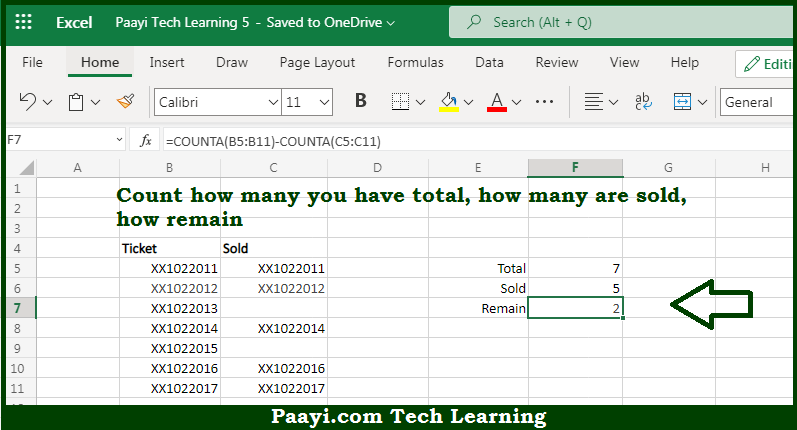# Learn How to COUNT Sold and Remaining Items in Microsoft Excel

Written by | 0 Comments | 366 Views

In this article, you will learn how to COUNT various things in Microsoft Excel using a single or combination of functions and its purpose. You will also get to know how to COUNT the Sold and Remaining Items and see the generic formula.

COUNT Sold and Remaining Items in Microsoft Excel

The main purpose of this formula is to count how many you have in total, how many are sold, how many remaining, etc. Here we will learn how to count sold and remaining items in Microsoft Excel. That implies, with the help of a formula based on the COUNTA function you can able to count how many you have total, how many are sold, how to remain, etc. This can be very important in the case where you are selling any kind of tickets, entries, passes, seats, or anything where you maintain and track an inventory of items sold So, with the help of this formula, you can able to count sold and remaining items.

General Formula to COUNT Sold and Remaining Items

=COUNTA(range1)-COUNTA(range2)

The Explanation for the COUNT Sold and Remaining ItemsSo we know that with the help of the given formula above you can able to count how many you have total, how many are sold, how many remaining, etc. Here we will learn how to count sold and remaining items in Microsoft Excel. As we know that the COUNTA function counts non-blank cells having numbers or text. The first COUNTA counts non-blank cells in the range B5:B11 and returns the number. The second COUNTA function does the same with the range C5:C11 and returns the numeric value since there are some non-blank cells in that range So, with the help of this formula, you can able to count how many you have total, how many are sold, how many remaining, etc.